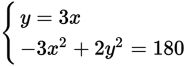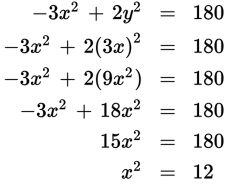# SAT Math Multiple Choice Question 409: Answer and Explanation

### Test Information

Question: 409

4.If (x, y) is a solution to the system of equations above, what is the value of x2?

• A. 12
• B. 20
• C. 60
• D. 144

Explanation:

A

Difficulty: Medium